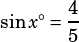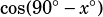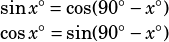Test 1 Section 3 number 19. Thanks!

You don’t need to draw to solve this one, but I think it’s often helpful to start a trig question by drawing a right triangle.SOH-CAH-TOA tells you that if, then the 4 must be across from the angle measuring x degrees, and the 5 must be the hypotenuse. If you’re up to speed with your Pythagorean triples, you also know that the other leg must have a length of 3.

Now, here’s the tricky part. The question asks for the value of. How the heck do we find that? Well, remember that in a right triangle, the two angles that aren’t the right angle must add up to 90º. So this question is really asking you for the cosine of the other angle in the right triangle we’ve drawn!What’s the cosine of that angle? Well, refer to SOH-CAH-TOA again. The cosine is the Adjacent leg over the Hypotenuse—that’s.

This is actually an identity worth remembering in case you should encounter a similar question on your test.